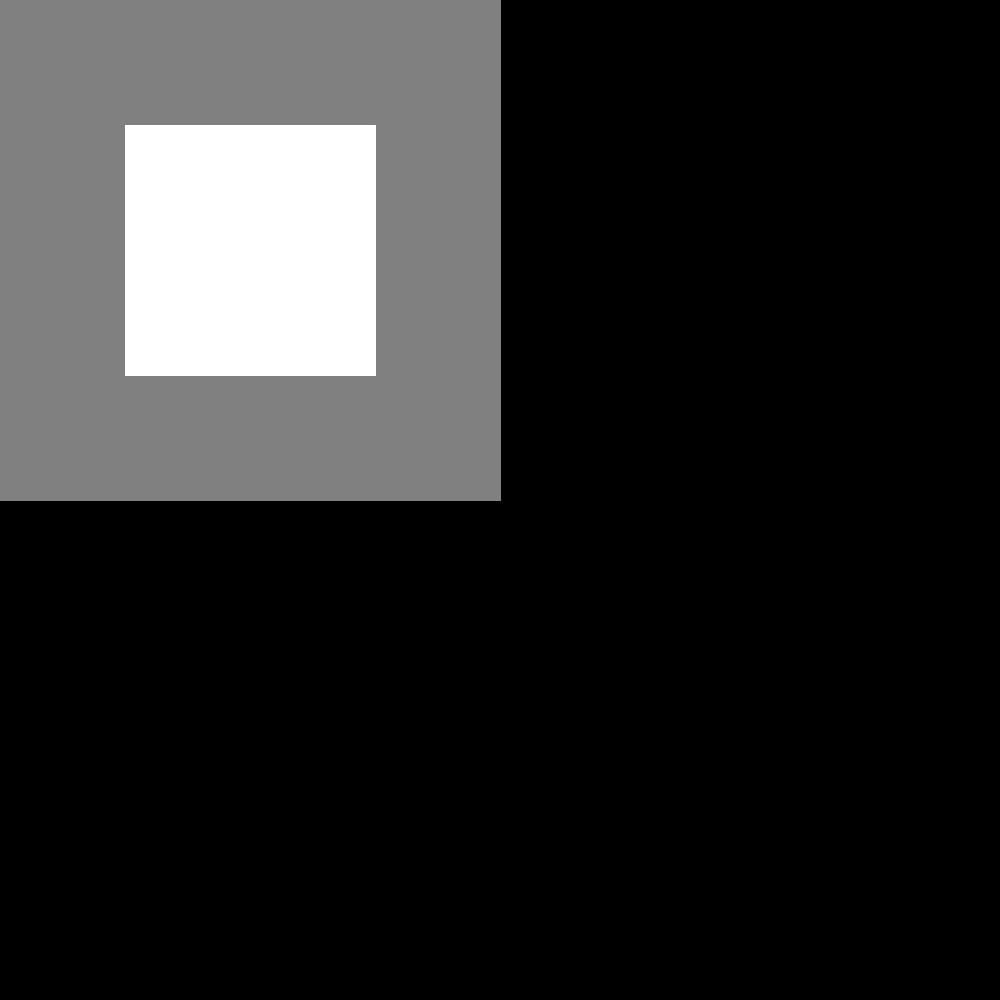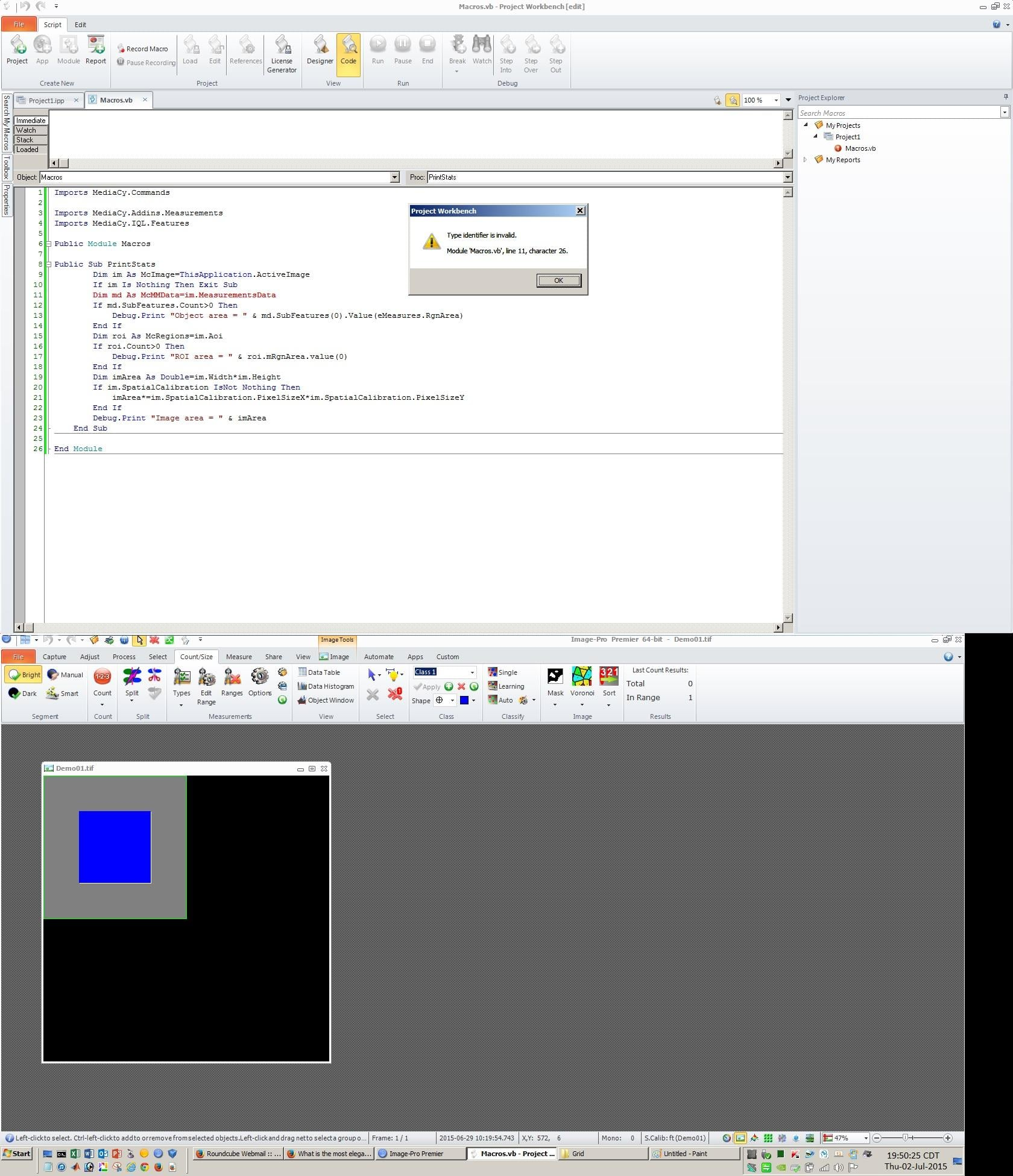What is the most elegant way to extract TOTAL OBJECT AREA, TOTAL ROI AREA, and TOTAL IMAGE AREA?

All --

What is the most elegant way to extract TOTAL OBJECT AREA, TOTAL ROI AREA, and TOTAL IMAGE AREA?

I the example image attached as

Demo01.tif

he following should be true

1 SQUARE PIXEL = 1 SQUARE FOOT
TOTAL OBJECT (WHITE) AREA = 63001 SQ FT
TOTAL ROI (GRAY and WHITE) AREA = 251001 SQ FT
TOTAL IMAGE (BLACK, GRAY, and WHITE) AREA = 1000000.00 SQ FT

What is the most elegant way (fewest lines of code required) to extract the three AREA MEASUREMENTS above from Demo01.tif?

Thanks.

-- Matt

• edited June 2015 Accepted Answer
Hi Matt,

Object and ROI measurements are reported in calibrated units, you have to calculate image area using calibration parameters. Here is a sample macro:

Imports MediaCy.IQL.Features

Public Module Macros

Public Sub PrintStats
Dim im As McImage=ThisApplication.ActiveImage
If im Is Nothing Then Exit Sub
Dim md As McMMData=im.MeasurementsData
If md.SubFeatures.Count>0 Then
Debug.Print "Object area = " & md.SubFeatures(0).Value(eMeasures.RgnArea)
End If
Dim roi As McRegions=im.Aoi
If roi.Count>0 Then
Debug.Print "ROI area = " & roi.mRgnArea.value(0)
End If
Dim imArea As Double=im.Width*im.Height
If im.SpatialCalibration IsNot Nothing Then
imArea*=im.SpatialCalibration.PixelSizeX*im.SpatialCalibration.PixelSizeY
End If
Debug.Print "Image area = " & imArea
End Sub

End Module

Yuri

• Here is the image (2nd try).

• Here is the image (3rd try).
•The FORUM would not let me upload the TIF image with the PREMIER CALIBRATION, ROI, and OBJECT.

Here is a JPG of the DEMO IMAGE.

Thanks.

-- Matt

• Yuri --

I'm sorry it took me so long to respond and thank you for addressing my question and providing the code sample.

The code you provided looks like it addresses these measurements perfectly for

1 OBJECT in
1 ROI in a
1 LAYER IMAGE

Two things . . .

1)

When I paste your code into the MACROS.VB of a CLEAN PROJECT, I get an error when I try to load the PROJECT.

The error is documented within the JPG FILE I have (hopefully) attached.

Imports MediaCy.IQL.Operators
and
Imports MediaCy.IQL.Operators.ImageOperators
to the IMPORTS SECTION because I found

*-*-*-*-*-*-*-*-*-*-*-*-

Image-Pro Premier Automation Help
MeasurementsData Method (image)

Automation Reference ► MediaCy.IQL.Operators ► ImageOperators ► MeasurementsData(McImage)  Visual Basic
Declaration Syntax
Visual Basic

<ExtensionAttribute> _
Public Shared Function MeasurementsData ( _
image As McImage _
) As Object

*-*-*-*-*-*-*-*-*-*-*-*-

but this did not resolve the problem.

2)

Is there an elegant way to "scale" this up to get the TOTAL OBJECT AREAS and TOTAL ROI AREAS in an image with

X OBJECTs in
Y ROIs

without looping through the "individual" OBJECTS and ROIS?

I imagine there is a way to strategically add.SUM in a couple of places (and probably delete the (0)s) that would make this happen but I need to get the basic code working first.

Thanks again.

-- Matt• Yuri --

The above code works great when embedded within an existing project.

I'd like to follow up to know how to figure out which IMPORT is missing and generating the error in the CLEAN PROJECT though.

Thanks again.

-- Matt